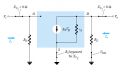# Simple Small Signal Fixed-Bias

#### MHR-Love

Joined Nov 15, 2010
10
Hi guys, in the simple fixed-bias amplifier shown Herewith its equivalent circuit,Why is the input voltage Vi equal to the gate-to-source voltage Vgs when we find the gain Av=Vo/Vi= (-gm*Vgs*(rd||Rd))/Vi, so Vgs=Vi and then Av will be =-gm(rd||Rd) as Vgs and Vi cancel each other???

Previously Vgs was equal to Vgg because there is no current the gate, but after we short circuited the sources and the capacitors to draw the equivalent circuit, Vi became = Vgs.

If it's like that, then we can say that Vi=Vgs=Gdd which is DC not AC?
Thnx very much

#### MHR-Love

Joined Nov 15, 2010
10
Hi guys, in the simple fixed-bias amplifier shown Here
View attachment 24630
with its equivalent circuit,
View attachment 24631
Why is the input voltage Vi equal to the gate-to-source voltage Vgs?? The gain Av=Vo/Vi= (-gm*Vgs*(rd||Rd))/Vi, then when we equate Vgs=Vi, Av becomes =-gm(rd||Rd) as Vgs and Vi cancel each other???

Previously Vgs was equal to Vgg because there is no gate current, but after we short circuit the sources and the capacitors to draw the equivalent circuit, Vi becomes = Vgs.

If it's like that, then we can say that Vi=Vgs=Gdd which is DC not AC?
Thnx very much

#### t_n_k

Joined Mar 6, 2009
5,455
It's true that for the ac case vi=vgs.

The AC and DC values of Vgs will be different. The DC (biased) value of Vgs will be something close to -2V. Depends on the gate leakage current. The small signal AC value does not include the DC bias for analytical purposes. For a given bias condition the small signal gain is explicitly determined by the small signal gain equation you gave.

#### MHR-Love

Joined Nov 15, 2010
10
It's true that for the ac case vi=vgs.

The AC and DC values of Vgs will be different. The DC (biased) value of Vgs will be something close to -2V. Depends on the gate leakage current. The small signal AC value does not include the DC bias for analytical purposes. For a given bias condition the small signal gain is explicitly determined by the small signal gain equation you gave.
but gm*Vgs=Id where Vgs is the dc value which is around -2V, now Vi is the input AC value. As you said the AC and DC values of Vgs are different. In the gain equation we substituted Vi=Vgs, So how can Vgs, the dc value, in (gm*Vgs) cancel Vi, the AC value, in the denominator??
Thank u and sorry I'm new to Amplifiers.

#### t_n_k

Joined Mar 6, 2009
5,455
The gain equation you quote is the small signal AC gain equation. It does not and should not include any DC terms.

The small signal AC model is derived on the basis of a suitable DC bias condition or operating point being established in the amplifier circuit. The small signal AC model is also derived on the credible assumption that the small signal behaviour is linear over "small" perturbations about the circuit DC operating point. In fact the "small" variations can be relatively large and the model still works quite well - as long as the device is not driven significantly into saturation or cut-off.

Last edited:

#### t_n_k

Joined Mar 6, 2009
5,455
It's also worth reflecting on the voltages one would 'see' at the input and output. Since both input and output terminals are capacitively coupled to the rest of the amplifier neither will have a measurable DC component. If the capacitor values are assumed to be sufficiently large then their AC impedance at a typical input frequency may be considered as being negligible. In that case the AC values of Vi and Vgs would be effectively identical.

The AC input voltage is what causes the small variations about the DC value of Vgs. If the input is zero then there is no change in Vgs. In that case there is no change in the drain current and hence no change in the drain voltage. Therefore no change observed at the output terminal.

One needs to keep in mind that the amplifier AC and DC operation are mutually dependent but that once the DC conditions are correctly established the AC analysis can be done without reference to the DC bias conditions.

Last edited:

#### MHR-Love

Joined Nov 15, 2010
10
It's also worth reflecting on the voltages one would 'see' at the input and output. Since both input and output terminals are capacitively coupled to the rest of the amplifier neither will have a measurable DC component. If the capacitor values are assumed to be sufficiently large then their AC impedance at a typical input frequency may be considered as being negligible. In that case the AC values of Vi and Vgs would be effectively identical.

The AC input voltage is what causes the small variations about the DC value of Vgs. If the input is zero then there is no change in Vgs. In that case there is no change in the drain current and hence no change in the drain voltage. Therefore no change observed at the output terminal.

One needs to keep in mind that the amplifier AC and DC operation are mutually dependent but that once the DC conditions are correctly established the AC analysis can be done without reference to the DC bias conditions.
That explanation is very much enough and thank you for this nice information.
Take care,
with appreciation